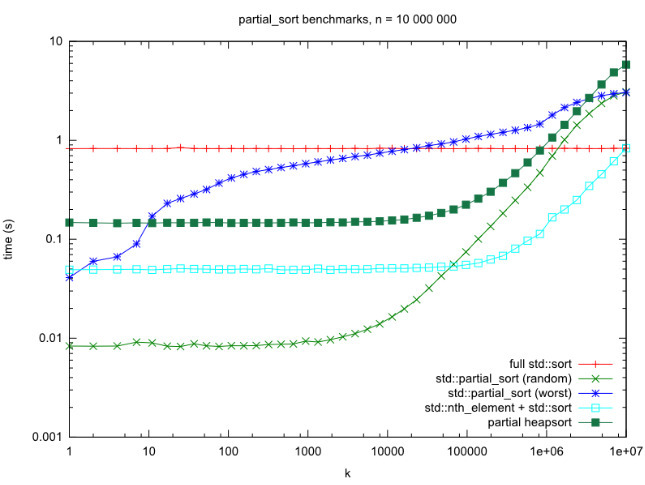# sort() vs. partial_sort() vs. nth_element() + sort() in C++ STL

In this article, we will discuss the difference between sort(), partial_sort(), and nth_element()+sort().

Below is the illustration of the above functions:

1. sort(): C++ STL provides a function sort() that sorts a list of element in O(N*log N) time. By default, sort() sorts an array in ascending order. Below is the program to illustrate sort():

 `// C++ program to illustrate the default behaviour ` `// of sort() in STL ` `#include ` `using` `namespace` `std; ` ` `  `// Driver Code ` `int` `main() ` `{ ` `    ``// Given array of elements ` `    ``int` `arr[] = { 1, 5, 8, 9, 6, 7, 3, 4, 2, 0 }; ` `    ``int` `n = ``sizeof``(arr) / ``sizeof``(arr); ` ` `  `    ``// Function sort() to sort the element of ` `    ``// the array in increasing order ` `    ``sort(arr, arr + n); ` ` `  `    ``// Print the array elements after sorting ` `    ``cout << ``"\nArray after sorting using "` `            ``"default sort is: \n"``; ` `    ``for` `(``int` `i = 0; i < n; ++i) { ` `        ``cout << arr[i] << ``" "``; ` `    ``} ` ` `  `    ``return` `0; ` `} `

Output:

```Array after sorting using default sort is :
0 1 2 3 4 5 6 7 8 9
```
2. partial_sort(): One of the variants of std::sort() is std::partial_sort(), which is used for sorting not the entire range, but only a sub-part of it. It rearranges the elements in the range [first, last), in such a way that the elements before middle are sorted in ascending order, whereas the elements after middle are left without any specific order.
Below is the program to illustrate partial_sort():

 `// C++ program to demonstrate the use of ` `// partial_sort() ` `#include ` `using` `namespace` `std; ` ` `  `// Driver Code ` `int` `main() ` `{ ` `    ``// Given array of elements ` `    ``vector<``int``> v = { 1, 3, 1, 10, 3, 3, 7, 7, 8 }; ` ` `  `    ``// Using std::partial_sort() to sort ` `    ``// first 3 elements ` `    ``partial_sort(v.begin(), v.begin() + 3, v.end()); ` ` `  `    ``// Displaying the vector after applying ` `    ``// partial_sort() ` `    ``for` `(``int` `ip : v) { ` `        ``cout << ip << ``" "``; ` `    ``} ` ` `  `    ``return` `0; ` `} `

Output:

```1 1 3 10 3 3 7 7 8
```

The complexity of partial_sort() is O(N*log K) where N is the number of elements in array and K is the number of elements between middle and start. The partial_sort() is faster than sort() if K is significantly smaller than N as partial_sort() will sort first K elements whereas sort() will sort all the N elements.
The worst-case O(N*log K) running time of partial_sort() doesn’t tell the whole story. Its average-case running time on random input is O(N + K*log K + K*(log K)*(log Nk)).
Because very little work is done to ignore each element that isn’t among the smallest K seen so far just a single comparison, the constant factor turns out to be difficult to beat for small K, even with an asymptotically better algorithm.

3. nth_element(): The nth_element() is an STL algorithm which rearranges the list in such a way such that the element at the nth position is the one which should be at that position if we sort the list.
It does not sort the list, just that all the elements, which precede the nth element are not greater than it, and all the elements which succeed it are not less than it.
Below is the program to illustrate nth_element():

 `// C++ program to demonstrate the use ` `// of std::nth_element ` `#include ` `using` `namespace` `std; ` ` `  `// Driver Code ` `int` `main() ` `{ ` `    ``// Given array v[] ` `    ``int` `v[] = { 3, 2, 10, 45, 33, 56, 23, 47 }; ` ` `  `    ``// Using nth_element with n as 5 ` `    ``nth_element(v, v + 4, v + 8); ` ` `  `    ``// Since, n is 5 so 5th element ` `    ``// should be sorted ` `    ``for` `(``int` `i = 0; i < 8; i++) ` `        ``cout << v[i] << ``" "``; ` ` `  `    ``return` `0; ` `} `

Output:

```3 2 10 23 33 56 45 47
```

Below is the benchmark comparison between three algorithms, varying N from 0 to 107(X-axis in the graph):The nth_element() + sort() solution is asymptotically fastest, and performs better results for larger K(which is most of them note the logarithmic scale). But it does lose to partial_sort() on random input for K < 70000, by up to a factor of 6.My Personal Notes arrow_drop_upCheck out this Author's contributed articles.

If you like GeeksforGeeks and would like to contribute, you can also write an article using contribute.geeksforgeeks.org or mail your article to contribute@geeksforgeeks.org. See your article appearing on the GeeksforGeeks main page and help other Geeks.

Please Improve this article if you find anything incorrect by clicking on the "Improve Article" button below.

Practice Tags :

Be the First to upvote.

Please write to us at contribute@geeksforgeeks.org to report any issue with the above content.